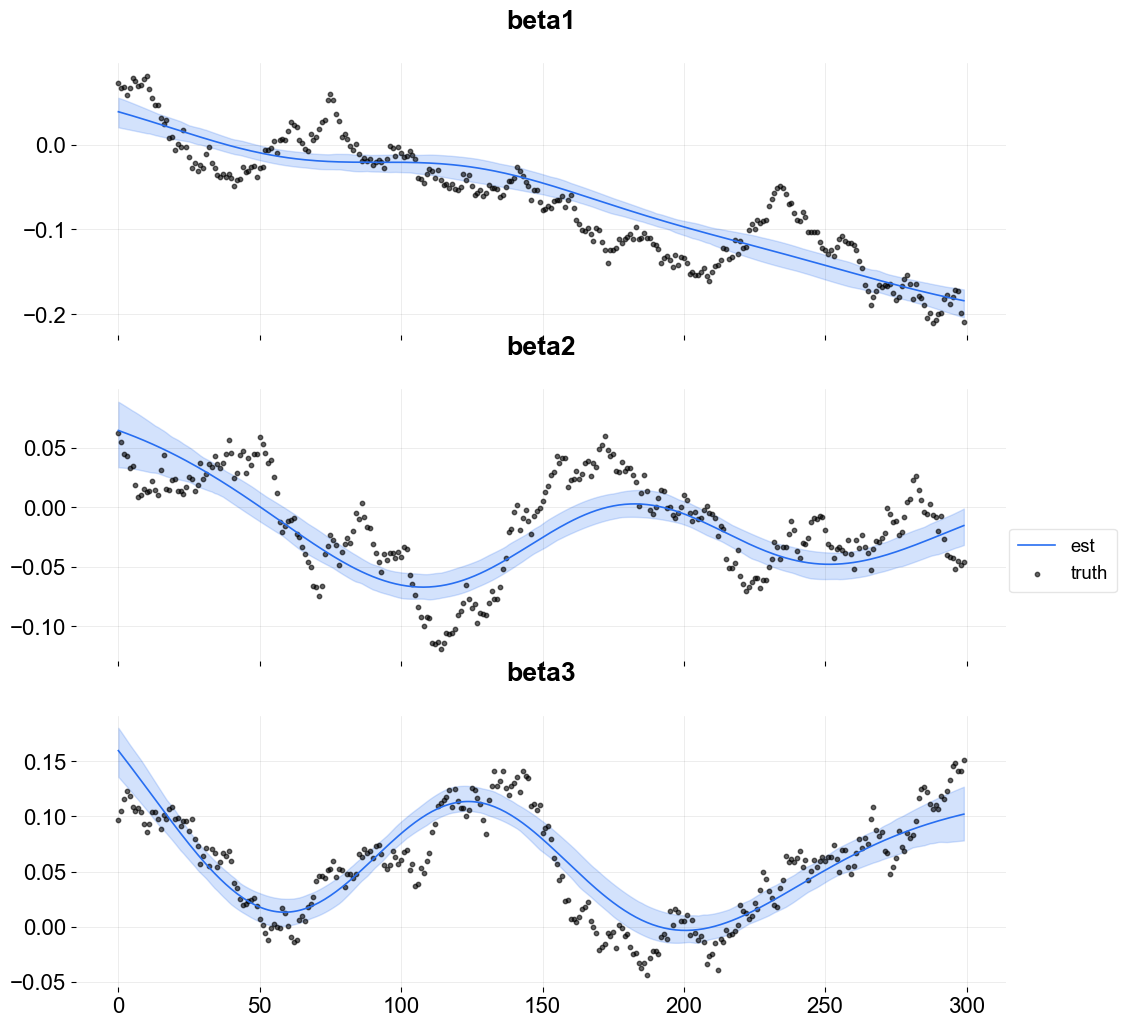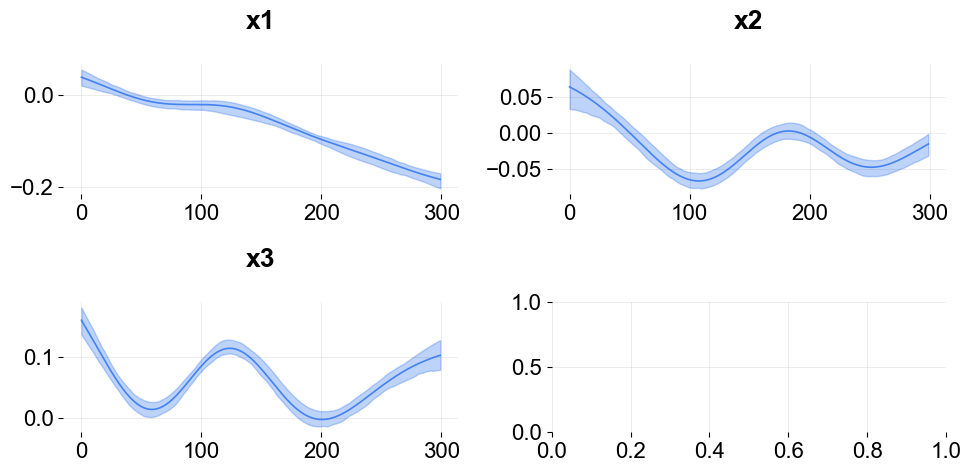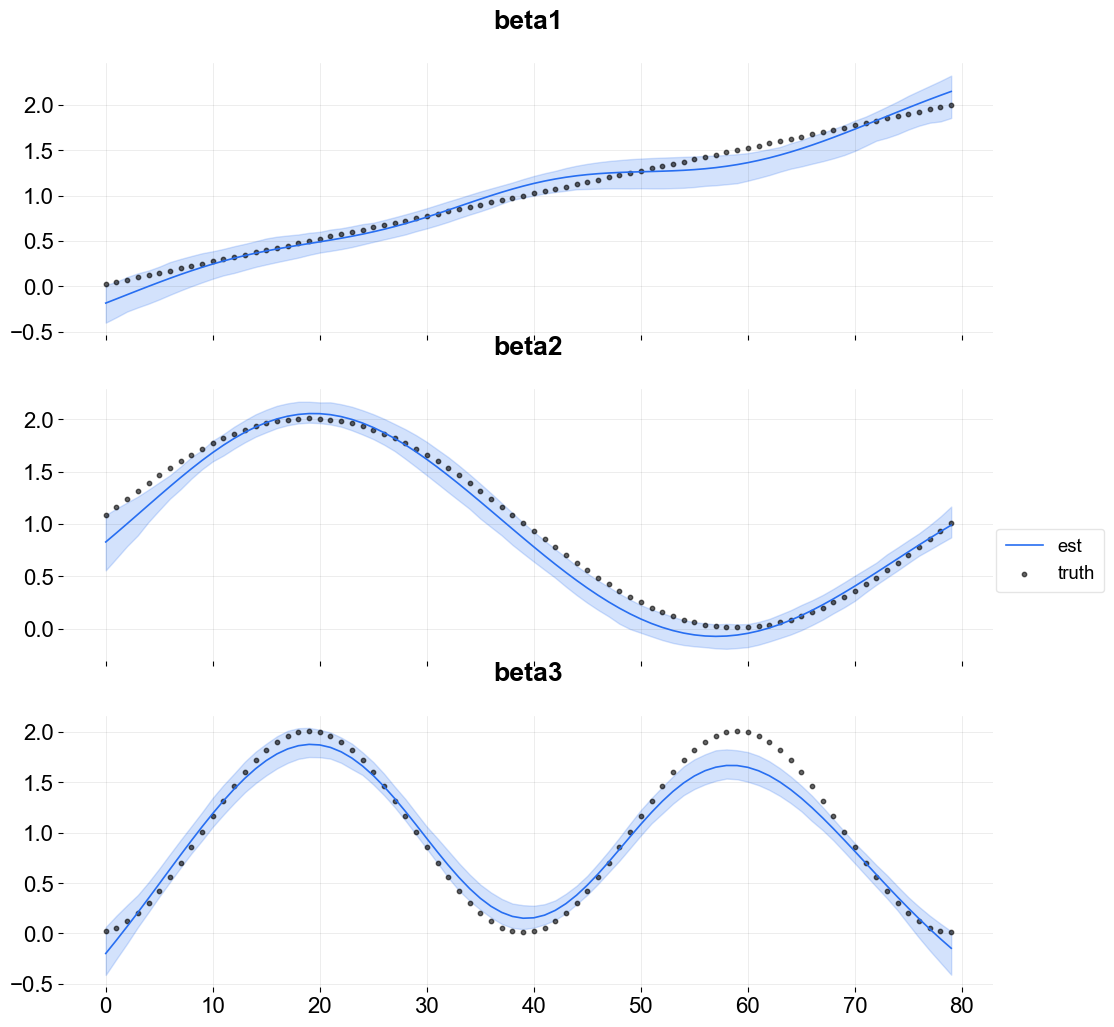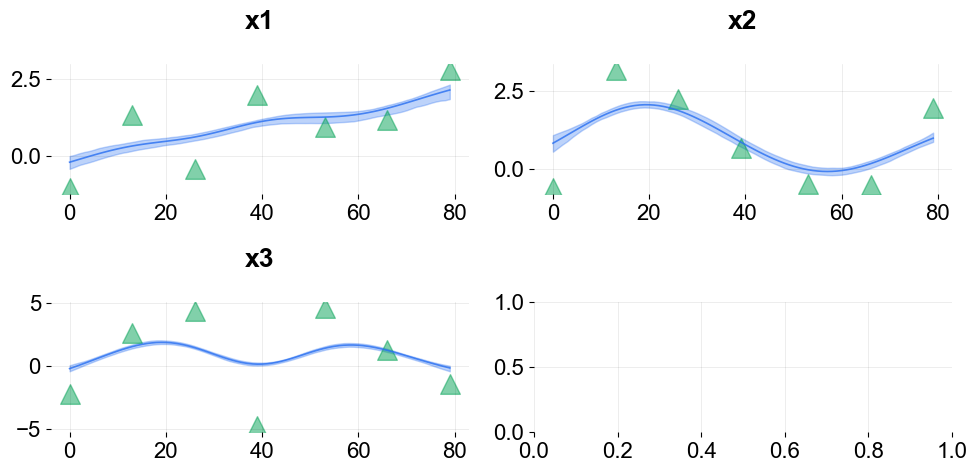# Kernel-based Time-varying Regression - Part II¶

The previous tutorial covered the basic syntax and structure of KTR (or so called BTVC); time-series data was fitted with a KTR model accounting for trend and seasonality. In this tutorial a KTR model is fit with trend, seasonality, and additional regressors. To summarize part 1, KTR considers a time-series as an additive combination of local-trend, seasonality, and additional regressors. The coefficients for all three components are allowed to vary over time. The time-varying of the coefficients is modeled using kernel smoothing of latent variables. This can also be an advantage of picking this model over other static regression coefficients models.

This tutorial covers:

1. KTR model structure with regression

2. syntax to initialize, fit and predict a model with regressors

3. visualization of regression coefficients

:

import pandas as pd
import numpy as np
from math import pi
import matplotlib.pyplot as plt

import orbit
from orbit.models import KTR
from orbit.diagnostics.plot import plot_predicted_components
from orbit.utils.plot import get_orbit_style
from orbit.constants.palette import OrbitPalette

%matplotlib inline
pd.set_option('display.float_format', lambda x: '%.5f' % x)
orbit_style = get_orbit_style()
plt.style.use(orbit_style);

:

print(orbit.__version__)

1.1.4


## Model Structure¶

This section gives the mathematical structure of the KTR model. In short, it considers a time-series ($$y_t$$) as the linear combination of three parts. These are the local-trend ($$l_t$$), seasonality (s_t), and regression ($$r_t$$) terms at time $$t$$. That is

$y_t = l_t + s_t + r_t + \epsilon_t, ~ t = 1,\cdots, T,$

where

• $$\epsilon_t$$s comprise a stationary random error process.

• $$r_t$$ is the regression component which can be further expressed as $$\sum_{i=1}^{I} {x_{i,t}\beta_{i, t}}$$ with covariate $$x$$ and coefficient $$\beta$$ on indexes $$i,t$$

For details of how on $$l_t$$ and $$s_t$$, please refer to Part I.

Recall in KTR, we express coefficients as

$B=K b^T$

where - coefficient matrix $$\text{B}$$ has size $$t \times P$$ with rows equal to the $$\beta_t$$ - knot matrix $$b$$ with size $$P\times J$$; each entry is a latent variable $$b_{p, j}$$. The $$b_j$$ can be viewed as the “knots” from the perspective of spline regression and $$j$$ is a time index such that $$t_j \in [1, \cdots, T]$$. - kernel matrix $$K$$ with size $$T\times J$$ where the $$i$$th row and $$j$$th element can be viewed as the normalized weight $$k(t_j, t) / \sum_{j=1}^{J} k(t_j, t)$$

In regression, we generate the matrix $$K$$ with Gaussian kernel $$k_\text{reg}$$ as such:

$$k_\text{reg}(t, t_j;\rho) = \exp ( -\frac{(t-t_j)^2}{2\rho^2} ),$$

where $$\rho$$ is the scale hyper-parameter.

## Data Simulation Module¶

In this example, we will use simulated data in order to have true regression coefficients for comparison. We propose two set of simulation data with three predictors each:

The two data sets are: - random walk - sine-cosine like

Note the data are random so it may be worthwhile to repeat the next few sets a few times to see how different data sets work.

### Random Walk Simulated Dataset¶

:

def sim_data_seasonal(n, RS):
""" coefficients curve are sine-cosine like
"""
np.random.seed(RS)
# make the time varing coefs
tau = np.arange(1, n+1)/n
data = pd.DataFrame({
'tau': tau,
'date': pd.date_range(start='1/1/2018', periods=n),
'beta1': 2 * tau,
'beta2': 1.01 + np.sin(2*pi*tau),
'beta3': 1.01 + np.sin(4*pi*(tau-1/8)),
'x1': np.random.normal(0, 10, size=n),
'x2': np.random.normal(0, 10, size=n),
'x3': np.random.normal(0, 10, size=n),
'trend': np.cumsum(np.concatenate((np.array(), np.random.normal(0, 0.1, n-1)))),
'error': np.random.normal(0, 1, size=n) #stats.t.rvs(30, size=n),#
})

data['y'] = data.x1 * data.beta1 + data.x2 * data.beta2 + data.x3 * data.beta3 + data.error
return data

:

def sim_data_rw(n, RS, p=3):
""" coefficients curve are random walk like
"""
np.random.seed(RS)

# initializing coefficients at zeros, simulate all coefficient values
lev = np.cumsum(np.concatenate((np.array([5.0]), np.random.normal(0, 0.01, n-1))))
beta = np.concatenate(
[np.random.uniform(0.05, 0.12, size=(1,p)),
np.random.normal(0.0, 0.01, size=(n-1,p))],
axis=0)
beta = np.cumsum(beta, 0)

# simulate regressors
covariates = np.random.normal(0, 10, (n, p))

# observation with noise
y = lev + (covariates * beta).sum(-1) + 0.3 * np.random.normal(0, 1, n)

regressor_col = ['x{}'.format(pp) for pp in range(1, p+1)]
data = pd.DataFrame(covariates, columns=regressor_col)
beta_col = ['beta{}'.format(pp) for pp in range(1, p+1)]
beta_data = pd.DataFrame(beta, columns=beta_col)
data = pd.concat([data, beta_data], axis=1)

data['y'] = y
data['date'] = pd.date_range(start='1/1/2018', periods=len(y))

return data

:

rw_data = sim_data_rw(n=300, RS=2021, p=3)

:

x1 x2 x3 beta1 beta2 beta3 y date
0 14.02970 -2.55469 4.93759 0.07288 0.06251 0.09662 6.11704 2018-01-01
1 6.23970 0.57014 -6.99700 0.06669 0.05440 0.10476 5.35784 2018-01-02
2 9.91810 -6.68728 -3.68957 0.06755 0.04487 0.11624 4.82567 2018-01-03
3 -1.17724 8.88090 -16.02765 0.05849 0.04305 0.12294 3.63605 2018-01-04
4 11.61065 1.95306 0.19901 0.06604 0.03281 0.11897 5.85913 2018-01-05
5 7.31929 3.36017 -6.09933 0.07825 0.03448 0.10836 5.08805 2018-01-06
6 0.53405 8.80412 -1.83692 0.07467 0.01847 0.10507 4.59303 2018-01-07
7 -16.03947 0.27562 -22.00964 0.06887 0.00865 0.10749 1.26651 2018-01-08
8 -17.72238 2.65195 0.22571 0.07007 0.01008 0.10432 4.10629 2018-01-09
9 -7.39895 -7.63162 3.25535 0.07715 0.01498 0.09356 4.30788 2018-01-10

### Sine-Cosine Like Simulated Dataset¶

:

sc_data = sim_data_seasonal(n=80, RS=2021)

:

tau date beta1 beta2 beta3 x1 x2 x3 trend error y
0 0.01250 2018-01-01 0.02500 1.08846 0.02231 14.88609 1.56556 -14.69399 1.00000 -0.73476 1.01359
1 0.02500 2018-01-02 0.05000 1.16643 0.05894 6.76011 -0.56861 4.93157 1.07746 -0.97007 -1.00463
2 0.03750 2018-01-03 0.07500 1.24345 0.11899 -4.18451 -5.38234 -13.90578 1.19201 -0.13891 -8.80009
3 0.05000 2018-01-04 0.10000 1.31902 0.20098 -8.06521 9.01387 -0.75244 1.22883 0.66550 11.59721
4 0.06250 2018-01-05 0.12500 1.39268 0.30289 5.55876 2.24944 -2.53510 1.31341 -1.58259 1.47715
5 0.07500 2018-01-06 0.15000 1.46399 0.42221 -7.05504 12.77788 14.25841 1.25911 -0.98049 22.68806
6 0.08750 2018-01-07 0.17500 1.53250 0.55601 11.30858 6.29269 7.82098 1.23484 -0.53751 15.43357
7 0.10000 2018-01-08 0.20000 1.59779 0.70098 6.45002 3.61891 16.28098 1.13237 -1.32858 17.15636
8 0.11250 2018-01-09 0.22500 1.65945 0.85357 1.06414 36.38726 8.80457 1.02834 0.87859 69.01607
9 0.12500 2018-01-10 0.25000 1.71711 1.01000 4.22155 -12.01221 8.43176 1.00649 -0.22055 -11.27534

## Fitting a Model with Regressors¶

The metadata for simulated data sets.

:

# num of predictors
p = 3
regressor_col = ['x{}'.format(pp) for pp in range(1, p + 1)]
response_col = 'y'
date_col='date'


As in Part I KTR follows sklearn model API style. First an instance of the Orbit class KTR is created. Second fit and predict methods are called for that instance. Besides providing meta data such response_col, date_col and regressor_col, there are additional args to provide to specify the estimator and the setting of the estimator. For details, please refer to other tutorials of the Orbit site.

:

ktr = KTR(
response_col=response_col,
date_col=date_col,
regressor_col=regressor_col,
prediction_percentiles=[2.5, 97.5],
seed=2021,
estimator='pyro-svi',
)


Here predict has the additional argument decompose=True. This returns the compponents ($$l_t$$, $$s_t$$, and $$r_t$$) of the regression along with the prediction.

:

ktr.fit(df=rw_data)

2023-01-20 18:01:50 - orbit - INFO - Optimizing (PyStan) with algorithm: LBFGS.
2023-01-20 18:01:51 - orbit - INFO - Using SVI (Pyro) with steps: 301, samples: 100, learning rate: 0.1, learning_rate_total_decay: 1.0 and particles: 100.
2023-01-20 18:01:51 - orbit - INFO - step    0 loss = 3107.7, scale = 0.091353
INFO:orbit:step    0 loss = 3107.7, scale = 0.091353
2023-01-20 18:01:51 - orbit - INFO - step  100 loss = 310.04, scale = 0.051308
INFO:orbit:step  100 loss = 310.04, scale = 0.051308
2023-01-20 18:01:52 - orbit - INFO - step  200 loss = 311.32, scale = 0.052509
INFO:orbit:step  200 loss = 311.32, scale = 0.052509
2023-01-20 18:01:53 - orbit - INFO - step  300 loss = 306.77, scale = 0.052749
INFO:orbit:step  300 loss = 306.77, scale = 0.052749

:

date prediction_2.5 prediction prediction_97.5 trend_2.5 trend trend_97.5 regression_2.5 regression regression_97.5
0 2018-01-01 5.25560 6.31047 7.36121 4.05561 5.12412 6.23188 0.89832 1.20754 1.50318
1 2018-01-02 3.32682 4.28862 5.21764 4.17170 5.08803 6.05128 -1.01434 -0.80619 -0.54898
2 2018-01-03 3.54542 4.67936 5.86222 4.12870 5.17765 6.38459 -0.81474 -0.50940 -0.12606
3 2018-01-04 1.99021 3.06280 4.10141 4.06234 5.10280 6.13881 -2.45662 -2.03059 -1.48406
4 2018-01-05 4.77952 5.61357 6.49165 4.18434 5.09533 5.98719 0.30557 0.55446 0.75344

## Visualization of Regression Coefficient Curves¶

The function get_regression_coefs to extract coefficients (they will have central credibility intervals if the argument include_ci=True is used).

:

coef_mid, coef_lower, coef_upper  = ktr.get_regression_coefs(include_ci=True)

:

coef_mid.head(5)

:

date x1 x2 x3
0 2018-01-01 0.03948 0.04946 0.15908
1 2018-01-02 0.03858 0.04848 0.15594
2 2018-01-03 0.03767 0.04749 0.15277
3 2018-01-04 0.03676 0.04648 0.14958
4 2018-01-05 0.03584 0.04545 0.14636

Because this is simulated data it is possible to overlay the estimate with the true coefficients.

:

fig, axes = plt.subplots(p, 1, figsize=(12, 12), sharex=True)

x = np.arange(coef_mid.shape)
for idx in range(p):
axes[idx].plot(x, coef_mid['x{}'.format(idx + 1)], label='est' if idx == 0 else "", color=OrbitPalette.BLUE.value)
axes[idx].fill_between(x, coef_lower['x{}'.format(idx + 1)], coef_upper['x{}'.format(idx + 1)], alpha=0.2, color=OrbitPalette.BLUE.value)
axes[idx].scatter(x, rw_data['beta{}'.format(idx + 1)], label='truth' if idx == 0 else "", s=10, alpha=0.6, color=OrbitPalette.BLACK.value)
axes[idx].set_title('beta{}'.format(idx + 1))

fig.legend(bbox_to_anchor = (1,0.5));To plot coefficients use the function plot_regression_coefs from the KTR class.

:

ktr.plot_regression_coefs(figsize=(10, 5), include_ci=True);These type of time-varying coefficients detection problems are not new. Bayesian approach such as the R packages Bayesian Structural Time Series (a.k.a BSTS) by Scott and Varian (2014) and tvReg Isabel Casas and Ruben Fernandez-Casal (2021). Other frequentist approach such as Wu and Chiang (2000).

For further studies on benchmarking coefficients detection, Ng, Wang and Dai (2021) provides a detailed comparison of KTR with other popular time-varying coefficients methods; KTR demonstrates superior performance in the random walk data simulation.

## Customizing Priors and Number of Knot Segments¶

To demonstrate how to specify the number of knots and priors consider the sine-cosine like simulated dataset. In this dataset, the fitting is more tricky since there could be some better way to define the number and position of the knots. There are obvious “change points” within the sine-cosine like curves. In KTR there are a few arguments that can leveraged to assign a priori knot attributes:

1. regressor_init_knot_loc is used to define the prior mean of the knot value. e.g. in this case, there is not a lot of prior knowledge so zeros are used.

2. The regressor_init_knot_scale and regressor_knot_scale are used to tune the prior sd of the global mean of the knot and the sd of each knot from the global mean respectively. These create a plausible range for the knot values.

3. The regression_segments defines the number of between knot segments (the number of knots - 1). The higher the number of segments the more change points are possible.

:

ktr = KTR(
response_col=response_col,
date_col=date_col,

regressor_col=regressor_col,
regressor_init_knot_loc= * len(regressor_col),
regressor_init_knot_scale=[10.0] * len(regressor_col),
regressor_knot_scale=[2.0] * len(regressor_col),
regression_segments=6,

prediction_percentiles=[2.5, 97.5],
seed=2021,
estimator='pyro-svi',
)
ktr.fit(df=sc_data)

2023-01-20 18:01:54 - orbit - INFO - Optimizing (PyStan) with algorithm: LBFGS.
INFO:orbit:Optimizing (PyStan) with algorithm: LBFGS.
2023-01-20 18:01:54 - orbit - INFO - Using SVI (Pyro) with steps: 301, samples: 100, learning rate: 0.1, learning_rate_total_decay: 1.0 and particles: 100.
INFO:orbit:Using SVI (Pyro) with steps: 301, samples: 100, learning rate: 0.1, learning_rate_total_decay: 1.0 and particles: 100.
2023-01-20 18:01:54 - orbit - INFO - step    0 loss = 828.02, scale = 0.10882
INFO:orbit:step    0 loss = 828.02, scale = 0.10882
2023-01-20 18:01:55 - orbit - INFO - step  100 loss = 340.58, scale = 0.87797
INFO:orbit:step  100 loss = 340.58, scale = 0.87797
2023-01-20 18:01:55 - orbit - INFO - step  200 loss = 266.67, scale = 0.37411
INFO:orbit:step  200 loss = 266.67, scale = 0.37411
2023-01-20 18:01:56 - orbit - INFO - step  300 loss = 261.21, scale = 0.43775
INFO:orbit:step  300 loss = 261.21, scale = 0.43775

:

<orbit.forecaster.svi.SVIForecaster at 0x2a6cd9190>

:

coef_mid, coef_lower, coef_upper  = ktr.get_regression_coefs(include_ci=True)
fig, axes = plt.subplots(p, 1, figsize=(12, 12), sharex=True)

x = np.arange(coef_mid.shape)
for idx in range(p):
axes[idx].plot(x, coef_mid['x{}'.format(idx + 1)], label='est' if idx == 0 else "", color=OrbitPalette.BLUE.value)
axes[idx].fill_between(x, coef_lower['x{}'.format(idx + 1)], coef_upper['x{}'.format(idx + 1)], alpha=0.2, color=OrbitPalette.BLUE.value)
axes[idx].scatter(x, sc_data['beta{}'.format(idx + 1)], label='truth' if idx == 0 else "", s=10, alpha=0.6, color=OrbitPalette.BLACK.value)
axes[idx].set_title('beta{}'.format(idx + 1))

fig.legend(bbox_to_anchor = (1, 0.5));Visualize the knots using the plot_regression_coefs function with with_knot=True.

:

ktr.plot_regression_coefs(with_knot=True, figsize=(10, 5), include_ci=True);There are more ways to define knots for regression as well as seasonality and trend (a.k.a levels). These are described in Part III